Optimal Parameters-based Geographical Detectors (OPGD) Model for Spatial Heterogeneity Analysis and Factor Exploration

2021-04-27

Current version: GD v1.10

Recommendation: Understanding Spatial Stratified Heterogeneity Using R

Citation for package GD

To cite GD R package in publications, please use:

Song, Y., Wang, J., Ge, Y. & Xu, C. (2020) “An optimal parameters-based geographical detector model enhances geographic characteristics of explanatory variables for spatial heterogeneity analysis: Cases with different types of spatial data”, GIScience & Remote Sensing. 57(5), 593-610. doi: 10.1080/15481603.2020.1760434.

Authors’ affiliations

Dr. Yongze Song

Research interests: Spatial statistics, sustainable infrastructure

Curtin University, Australia

1. Introduction to GD package

The model can be used to address following issues:

• Explore potential factors or explanatory variables from a spatial perspective.

• Explore potential interactive impacts of geogrpahical variables.

• Identify high-risk or low-risk regions from potential explanatory variables.

The GD package makes following steps fast and easy:

• It contains both supervised and unsupervised spatial data discretization methods, and the optimal spatial discretization method for continuous variables;

• It contains four primary functions of geographical detectors, including factor detector, risk detector, interaction detector and ecological detector;

• It can be used to compare size effects of spatial unit;

• It provides diverse visualizations of spatial analysis results;

• It contains detailed significance tests for spatial analysis in each step of geographical detectors.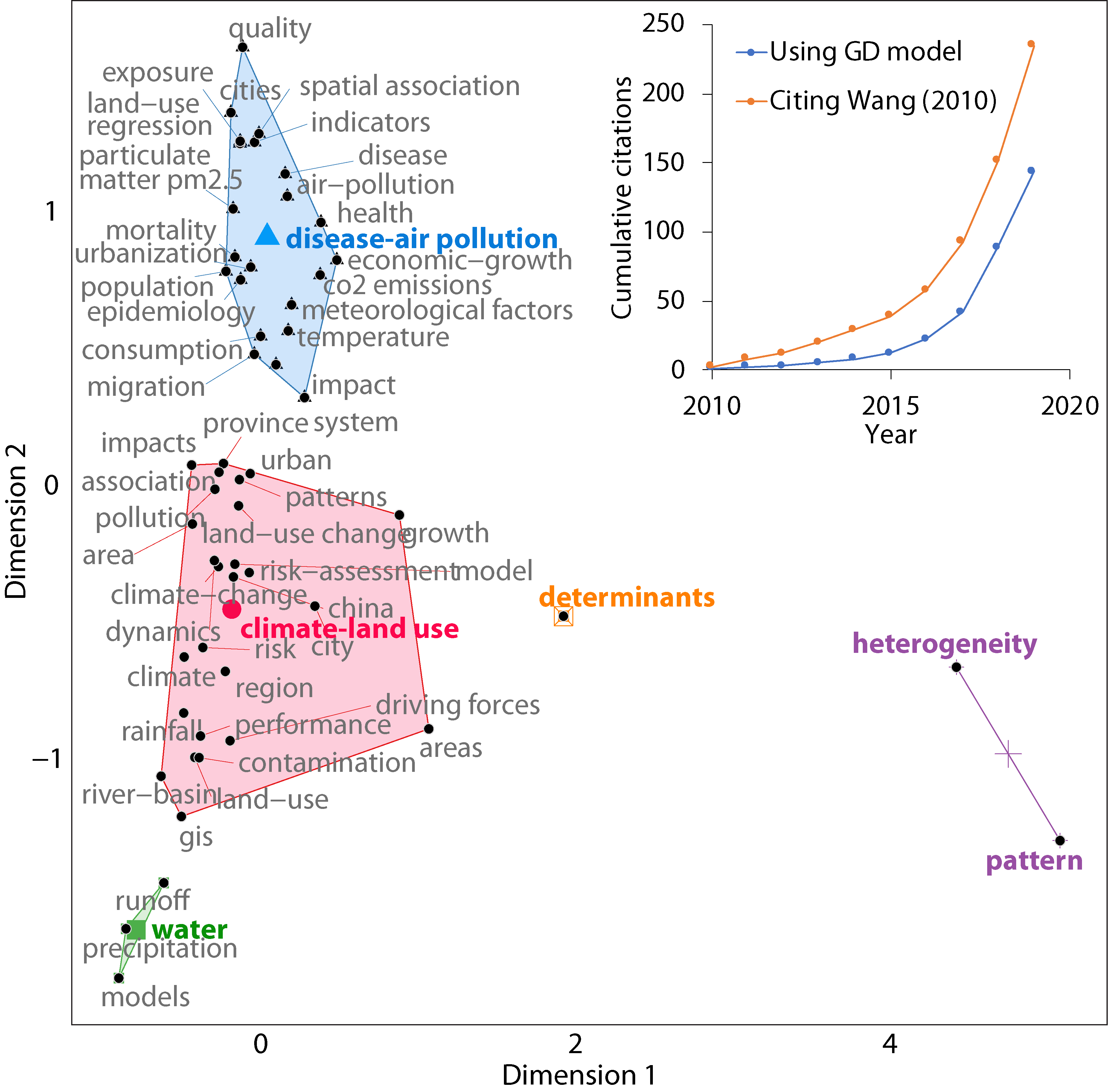Figure 1. Overview of global research using geographical detector model (cumulative citations were updated on June 2020). (Song et al. 2020)

2. Geographical detector model

Spatial stratified heterogeneity can be measured using geographical detectors (Wang et al. 2010, Wang et al. 2016).

Power of determinants is computed using a $$Q$$-statistic:

$Q=1-\displaystyle \frac{\sum_{j=1}^{M} N_{j} \sigma_{j}^2}{N \sigma^2}$

where $$N$$ and $$\sigma^2$$ are the number and population variance of observations within the whole study area, and $$N_{j}$$ and $$\sigma_{j}^2$$ are the number and population variance of observations within the $$j$$ th ($$j$$=1,…,$$M$$) sub-region of an explantory variable.

Please note that in R environment, sd and var functions are used for computing sample standard deviation and sample variance. If sample variance is used in the computation, the equation of $$Q$$-statistic can be converted to:

$Q=1-\displaystyle \frac{\sum_{j=1}^{M} (N_{j}-1) s_{j}^2}{(N-1) s^2}$

where $$s^2$$ and $$s_{j}^2$$ are sample variance of observations in the whole study area and in the $$j$$ th sub-region.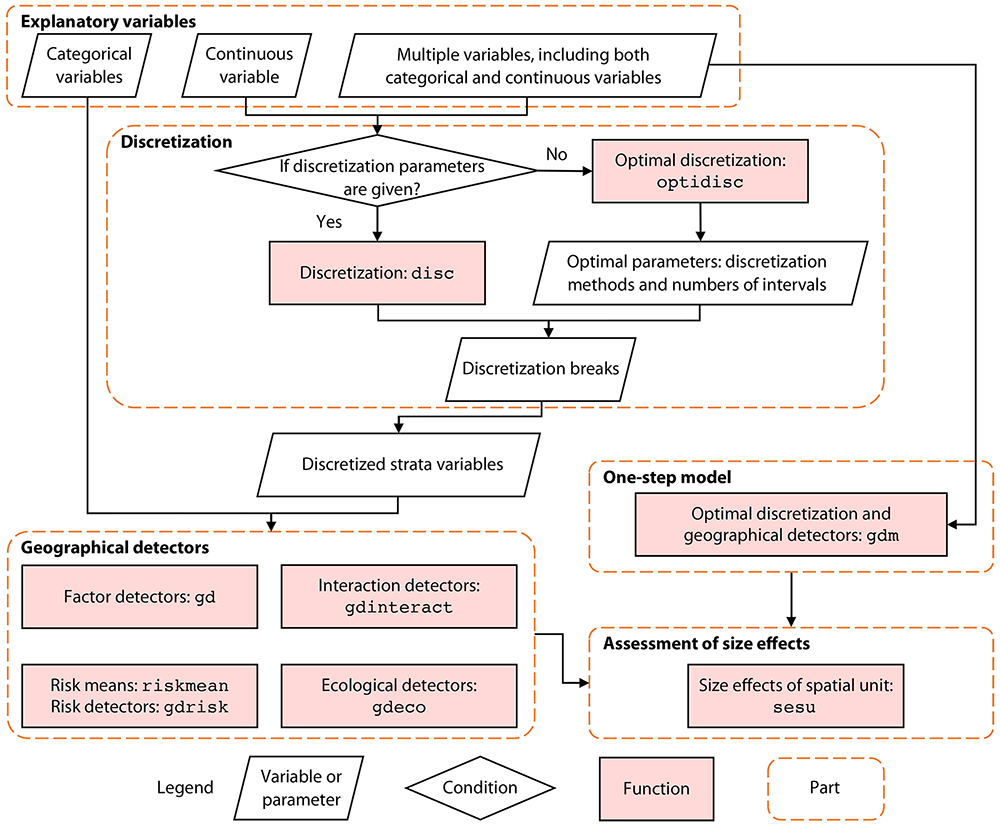Figure 2. General calculation process and relationships of functions in GD package (Song et al. 2020)

Further information can be found on the manual of GD package.

More applications of geographical detectors are listed on Geodetector website.

3. Spatial data discretization

Categorical variables are required for geographical detectors, so continuous variables should be discretized before modelling. GD package provides two options: discretization with given parameters, including discretization methods and numbers of intervals, and optimal discretization with a series of optional parameter combinations. Dataset ndvi_40 is used as an example for explanation.

install.packages("GD")
library("GD")
## This is GD 1.10.
##
## To cite GD in publications, please use:
##
## Song, Y., Wang, J., Ge, Y. & Xu, C. (2020) An optimal parameters-based geographical detector model enhances geographic characteristics of explanatory variables for spatial heterogeneity analysis: Cases with different types of spatial data, GIScience & Remote Sensing, 57(5), 593-610. doi: 10.1080/15481603.2020.1760434.
##
data("ndvi_40")
##   NDVIchange Climatezone Mining Tempchange Precipitation   GDP Popdensity
## 1    0.11599         Bwk    low    0.25598        236.54 12.55    1.44957
## 2    0.01783         Bwk    low    0.27341        213.55  2.69    0.80124
## 3    0.13817         Bsk    low    0.30247        448.88 20.06   11.49432

Discretization with given parameters: disc

## discretization methods: equal, natural, quantile (default), geometric, sd and manual
ds1 <- disc(ndvi_40$Tempchange, 4) ds1 plot(ds1) Further information can be found on the manual of GD package. Optimal discretization: optidisc ## set optional discretization methods and numbers of intervals discmethod <- c("equal","natural","quantile","geometric","sd") discitv <- c(4:7) ## optimal discretization odc1 <- optidisc(NDVIchange ~ Tempchange, data = ndvi_40, discmethod, discitv) odc1 plot(odc1)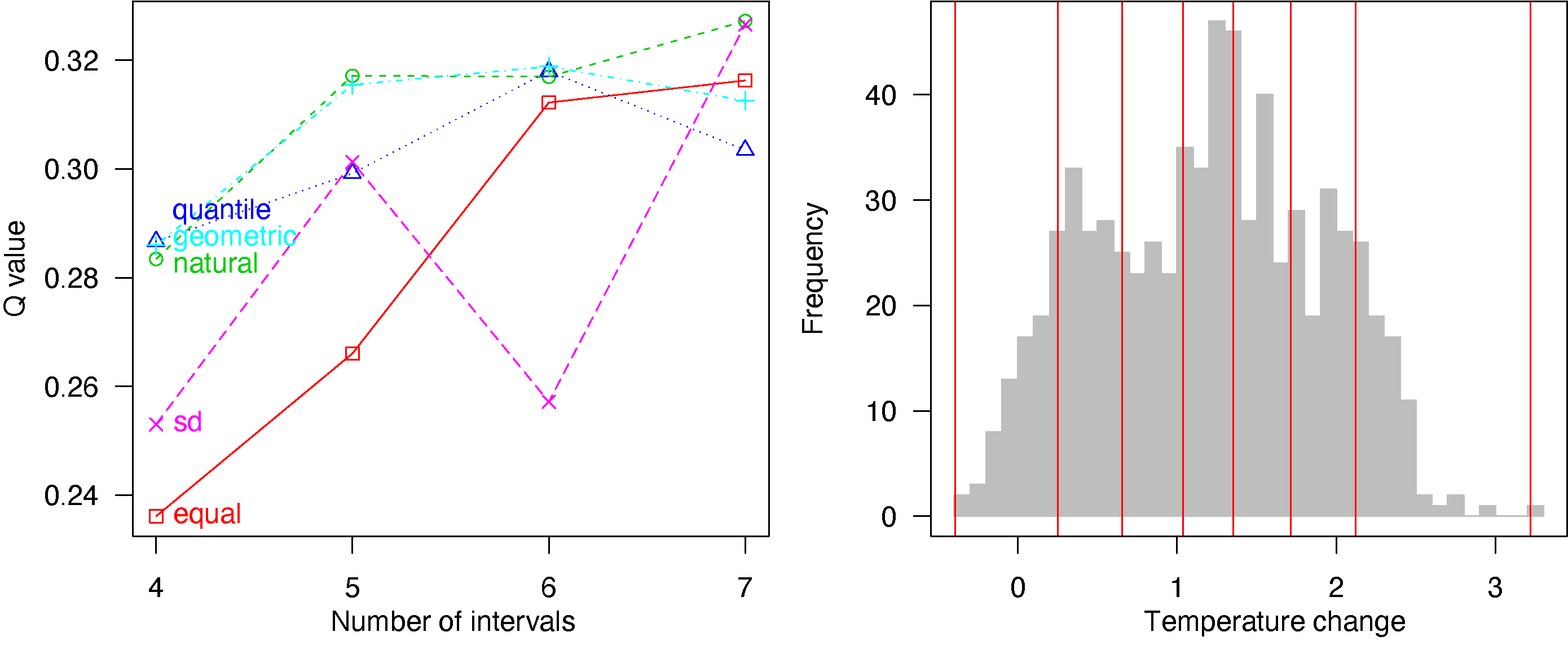Figure 3. Process and results of optimal spatial data discretization 4. Geographical detectors GD package provides two options for geographical detectors modelling: • four functions are performed step by step: gd for factor detector, riskmean and gdrisk for risk detector, gdinteract for interaction detector and gdeco for ecological detector; • optimal discretization and geographical detectors are performed using a one-step function gdm. Factor detector: gd ## a categorical explanatory variable g1 <- gd(NDVIchange ~ Climatezone, data = ndvi_40) g1 ## multiple categorical explanatory variables g2 <- gd(NDVIchange ~ ., data = ndvi_40[,1:3]) g2 plot(g2) ## multiple variables including continuous variables discmethod <- c("equal","natural","quantile","geometric","sd") discitv <- c(3:7) data.ndvi <- ndvi_40 data.continuous <- data.ndvi[, c(1, 4:7)] odc1 <- optidisc(NDVIchange ~ ., data = data.continuous, discmethod, discitv) # ~14s data.continuous <- do.call(cbind, lapply(1:4, function(x) data.frame(cut(data.continuous[, -1][, x], unique(odc1[[x]]$itv), include.lowest = TRUE))))
# add stratified data to explanatory variables
data.ndvi[, 4:7] <- data.continuous

g3 <- gd(NDVIchange ~ ., data = data.ndvi)
g3
plot(g3)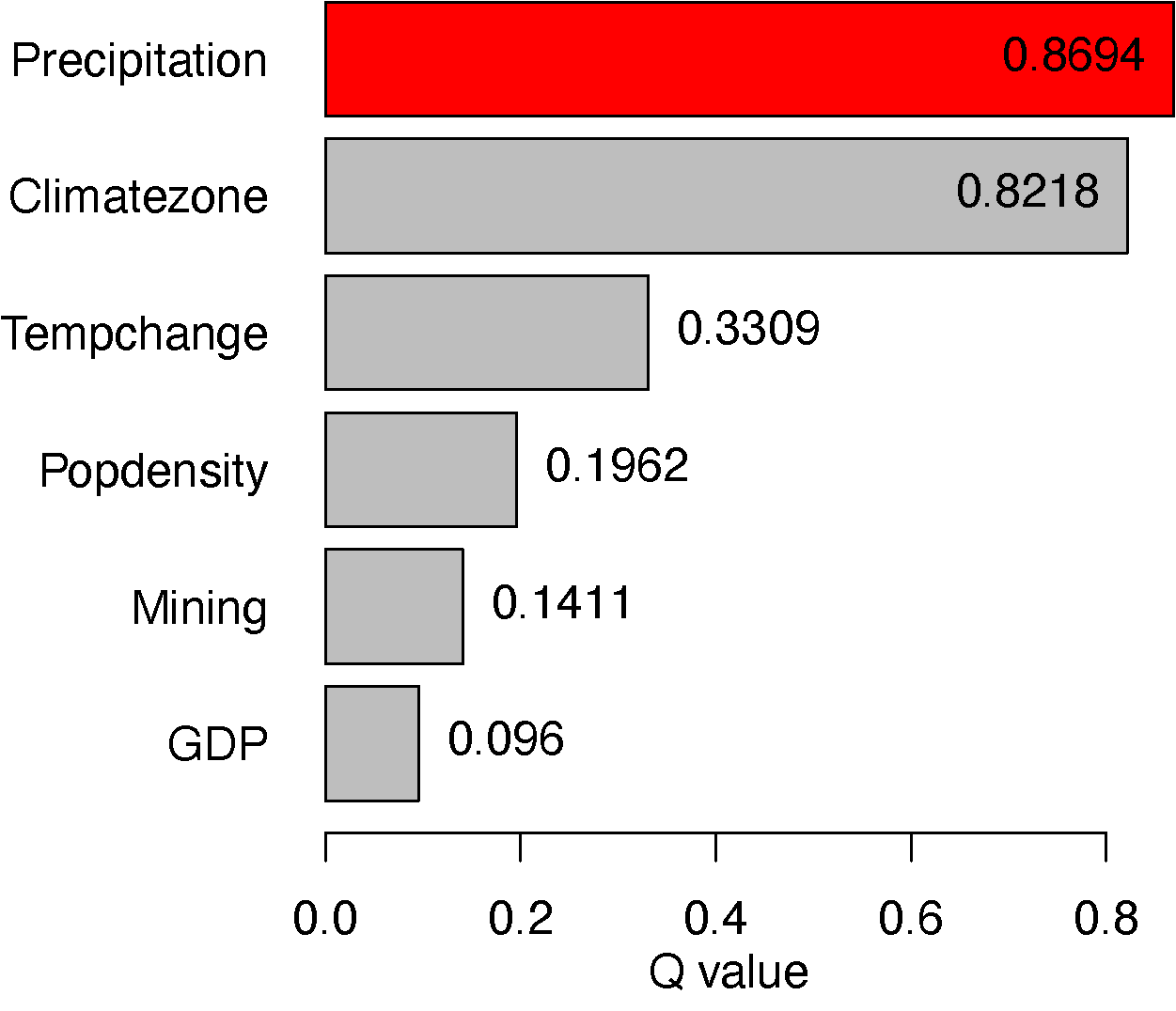Figure 4. Results of factor detector

Risk detector: riskmean and gdrisk

Risk mean values by variables:

## categorical explanatory variables
rm1 <- riskmean(NDVIchange ~ Climatezone + Mining, data = ndvi_40)
rm1
plot(rm1)
## multiple variables inclusing continuous variables
rm2 <- riskmean(NDVIchange ~ ., data = data.ndvi)
rm2
plot(rm2)

Risk matrix:

## categorical explanatory variables
gr1 <- gdrisk(NDVIchange ~ Climatezone + Mining, data = ndvi_40)
gr1
plot(gr1)
## multiple variables inclusing continuous variables
gr2 <- gdrisk(NDVIchange ~ ., data = data.ndvi)
gr2
plot(gr2)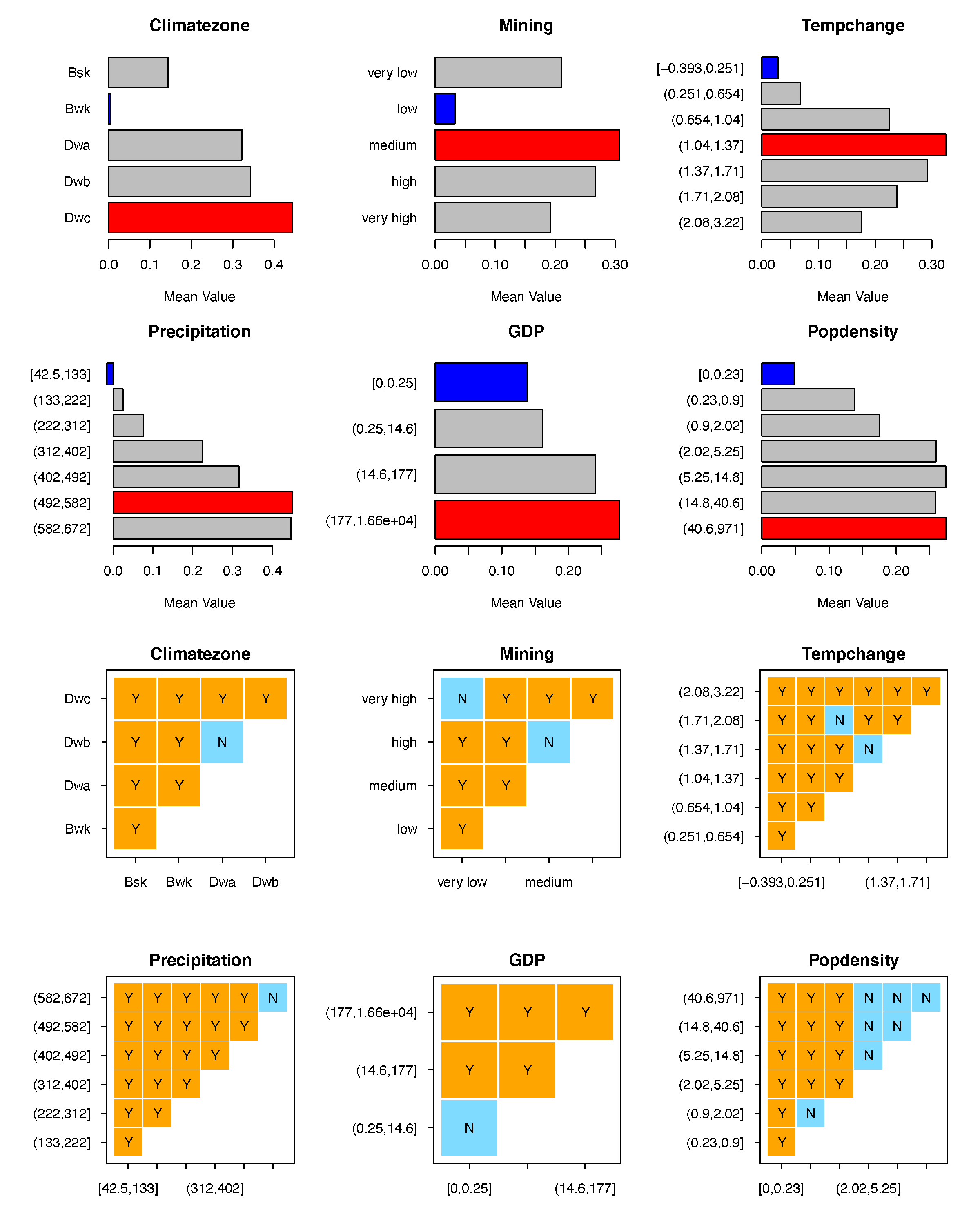Figure 5. Results of risk detector

Interaction detector: gdinteract

## categorical explanatory variables
gi1 <- gdinteract(NDVIchange ~ Climatezone + Mining, data = ndvi_40)
gi1
## multiple variables inclusing continuous variables
gi2 <- gdinteract(NDVIchange ~ ., data = data.ndvi)
gi2
plot(gi2)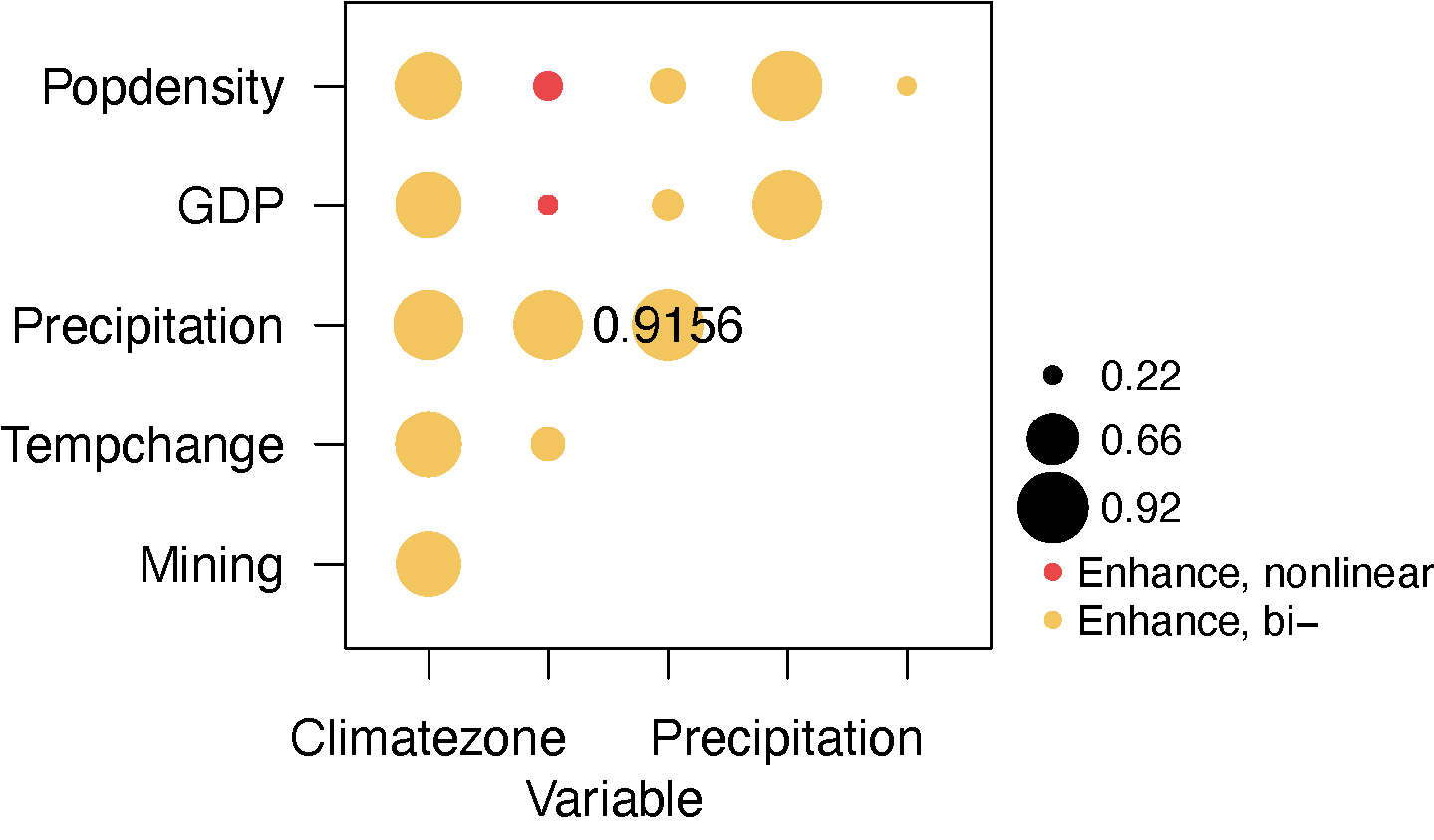Figure 6. Results of interaction detector

Ecological detector: gdeco

## categorical explanatory variables
ge1 <- gdeco(NDVIchange ~ Climatezone + Mining, data = ndvi_40)
ge1
## multiple variables inclusing continuous variables
gd3 <- gdeco(NDVIchange ~ ., data = data.ndvi)
gd3
plot(gd3)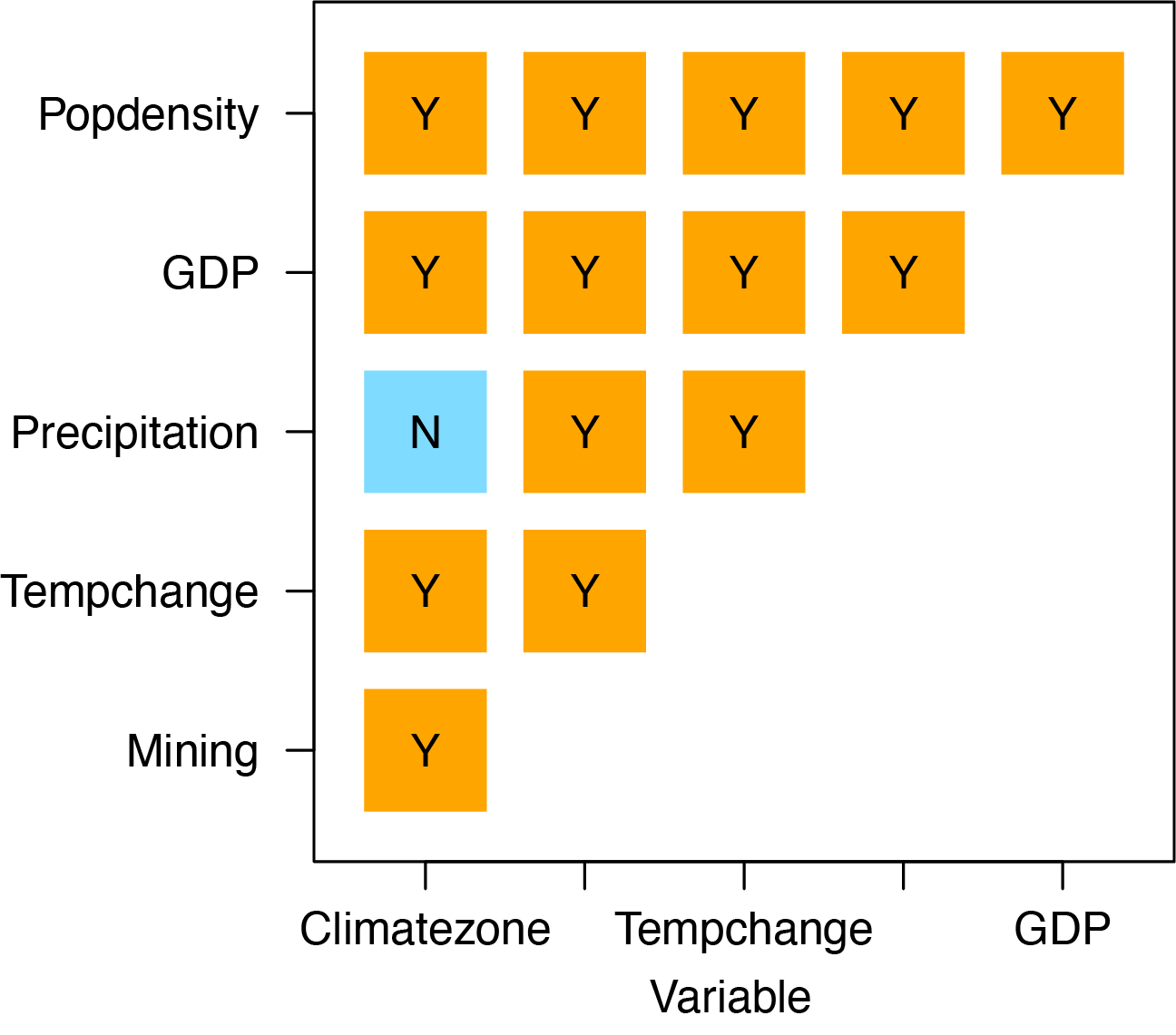Figure 7. Results of ecological detector

5. Comparison of size effects of spatial unit

ndvilist <- list(ndvi_20, ndvi_30, ndvi_40, ndvi_50)
su <- c(20,30,40,50) ## sizes of spatial units
## "gdm" function
gdlist <- lapply(ndvilist, function(x){
gdm(NDVIchange ~ Climatezone + Mining + Tempchange + GDP,
continuous_variable = c("Tempchange", "GDP"),
data = x, discmethod = "quantile", discitv = 6)
})
sesu(gdlist, su) ## size effects of spatial units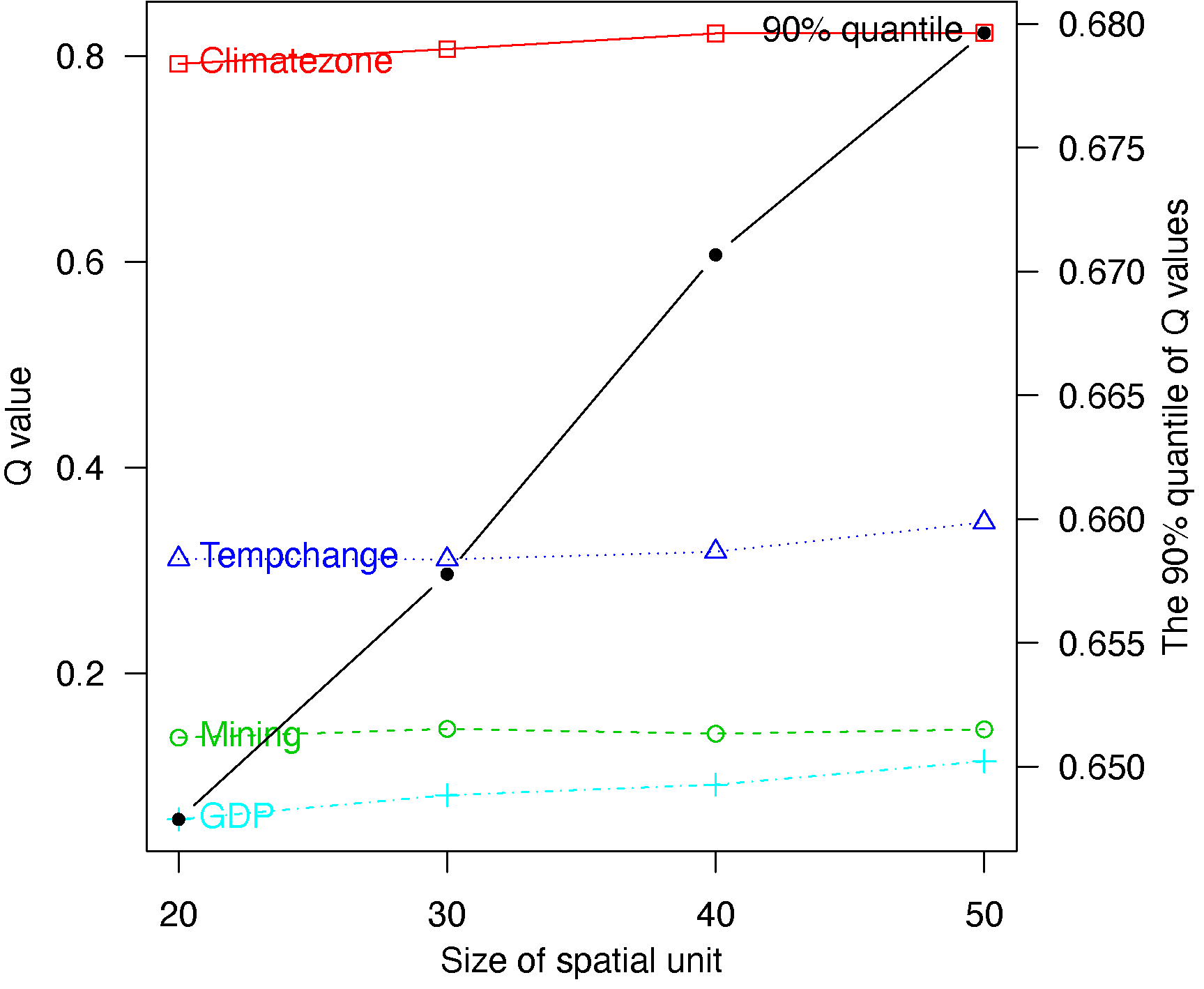Figure 8. Spatial scale effects

Reference

Song Y, Wang J, Ge Y and Xu C (2020) “An optimal parameters-based geographical detector model enhances geographic characteristics of explanatory variables for spatial heterogeneity analysis: Cases with different types of spatial data.” GIScience & Remote Sensing, 57(5), pp. 593-610. doi: 10.1080/15481603.2020.1760434.

Song Y, Wright G, Wu P, Thatcher D, McHugh T, Li Q, Li SJ and Wang X (2018). “Segment-Based Spatial Analysis for Assessing Road Infrastructure Performance Using Monitoring Observations and Remote Sensing Data”. Remote Sensing, 10(11), pp. 1696. doi: 10.3390/rs10111696.

Song Y, Wu P, Gilmore D and Li Q (2020). “A Spatial Heterogeneity-Based Segmentation Model for Analyzing Road Deterioration Network Data in Multi-Scale Infrastructure Systems.” IEEE Transactions on Intelligent Transportation Systems. doi: 10.1109/TITS.2020.3001193.

Wang J, Li X, Christakos G, Liao Y, Zhang T, Gu X and Zheng X (2010). “Geographical Detectors-Based Health Risk Assessment and its Application in the Neural Tube Defects Study of the Heshun Region, China.” International Journal of Geographical Information Science, 24(1), pp. 107-127. doi: 10.1080/13658810802443457.

Wang J, Zhang T and Fu B (2016). “A measure of spatial stratified heterogeneity.” Ecological Indicators, 67, pp. 250-256. doi: 10.1016/j.ecolind.2016.02.052.